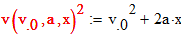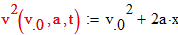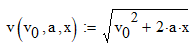cancel
Showing results for
Did you mean:
cancel
Showing results for
Did you mean:

Community Tip - You can change your system assigned username to something more personal in your community settings. X

## How do you enter a function that is squared2-Guest

## How do you enter a function that is squared

I am new to Mathcad, and am using Mathcad 15 for the moment. I am taking note as I read through my Physics book and want to enter the equaition for linear motion (single dimention). If I enter the following,andThe error, "A name is required here". I tried defining the above as:Which is equivalent, but, less desirable in my case. Is there no way to enter a function definition as a polynomial as I attempted?

Dave2-Guest
(To:DaveC)

Well, unfortunately, the board does not take a copy of an equation directly from Mathcad 15. The equations in my post should read:

2                2

v(v0,a,t):=v0 + 2*a*x

2      2

v(v0,a,t) :=v0 + 2*a*x                    <- Matcad display error "A name is required here."

and

----------------

/   2

v(vo,a,x) := -/ v0   + 2*a*x                 <- Mathcad accepts this

Dave